Скачать презентацию Why Should Historical Truth Matter to Teachers of

5a17a73fe81657cc59aa72fb04b51926.ppt

• Количество слайдов: 29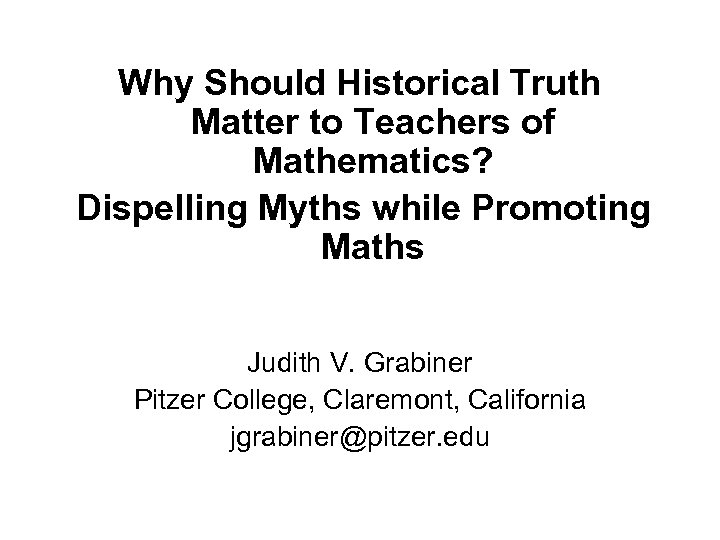Why Should Historical Truth Matter to Teachers of Mathematics? Dispelling Myths while Promoting Maths Judith V. Grabiner Pitzer College, Claremont, California [email protected] edu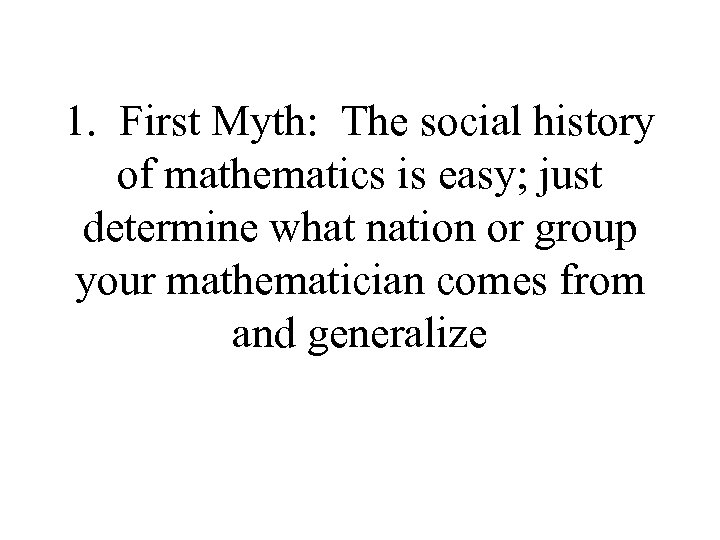1. First Myth: The social history of mathematics is easy; just determine what nation or group your mathematician comes from and generalize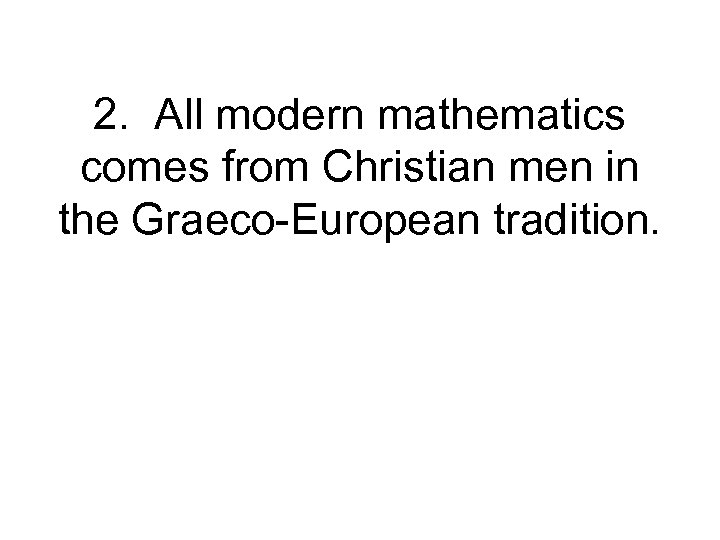2. All modern mathematics comes from Christian men in the Graeco-European tradition.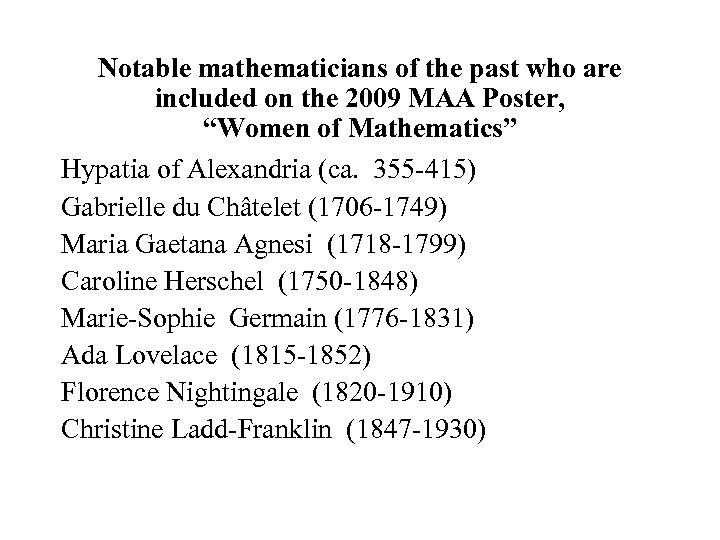Notable mathematicians of the past who are included on the 2009 MAA Poster, “Women of Mathematics” Hypatia of Alexandria (ca. 355 -415) Gabrielle du Châtelet (1706 -1749) Maria Gaetana Agnesi (1718 -1799) Caroline Herschel (1750 -1848) Marie-Sophie Germain (1776 -1831) Ada Lovelace (1815 -1852) Florence Nightingale (1820 -1910) Christine Ladd-Franklin (1847 -1930)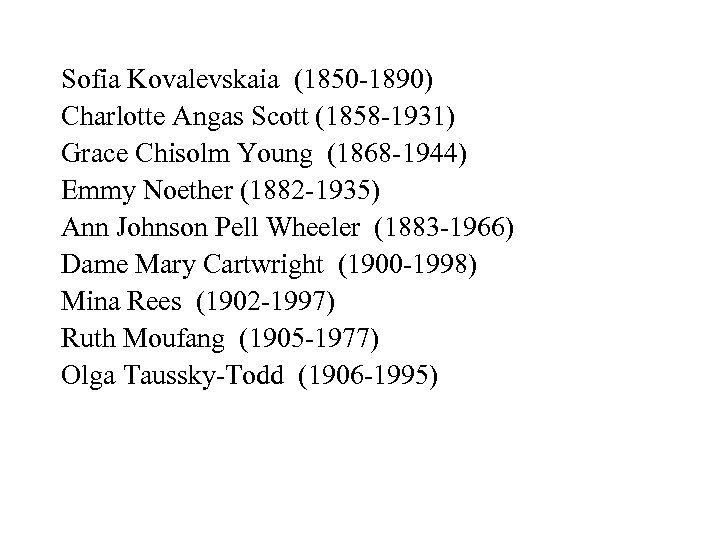Sofia Kovalevskaia (1850 -1890) Charlotte Angas Scott (1858 -1931) Grace Chisolm Young (1868 -1944) Emmy Noether (1882 -1935) Ann Johnson Pell Wheeler (1883 -1966) Dame Mary Cartwright (1900 -1998) Mina Rees (1902 -1997) Ruth Moufang (1905 -1977) Olga Taussky-Todd (1906 -1995)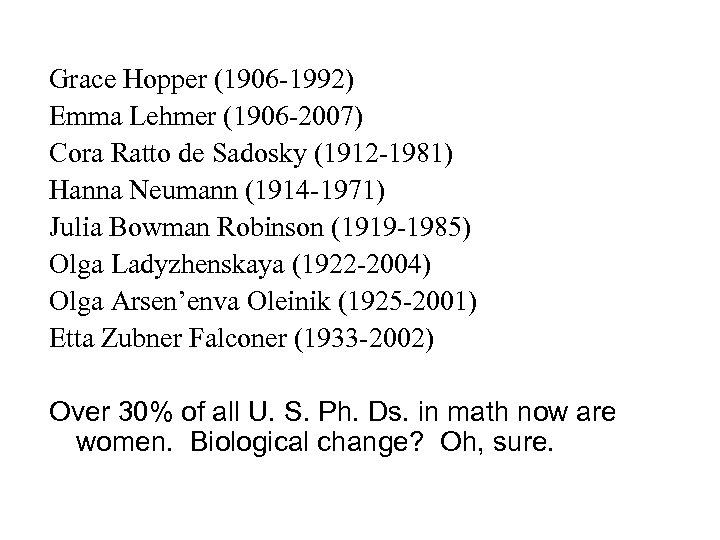Grace Hopper (1906 -1992) Emma Lehmer (1906 -2007) Cora Ratto de Sadosky (1912 -1981) Hanna Neumann (1914 -1971) Julia Bowman Robinson (1919 -1985) Olga Ladyzhenskaya (1922 -2004) Olga Arsen’enva Oleinik (1925 -2001) Etta Zubner Falconer (1933 -2002) Over 30% of all U. S. Ph. Ds. in math now are women. Biological change? Oh, sure.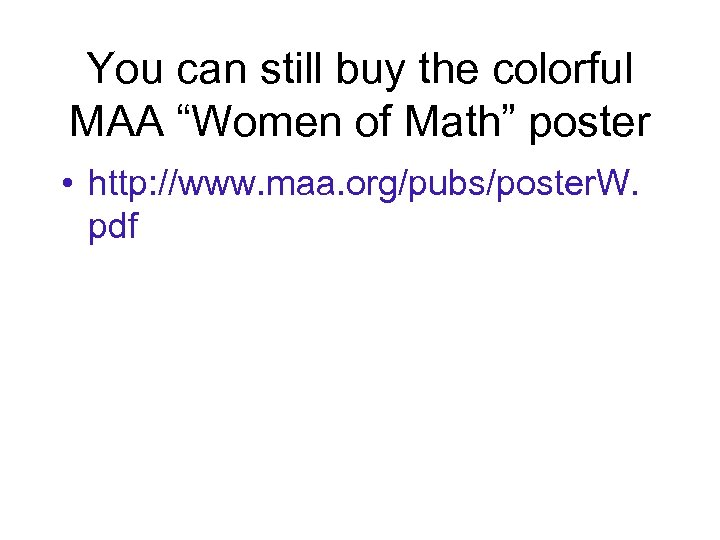You can still buy the colorful MAA “Women of Math” poster • http: //www. maa. org/pubs/poster. W. pdf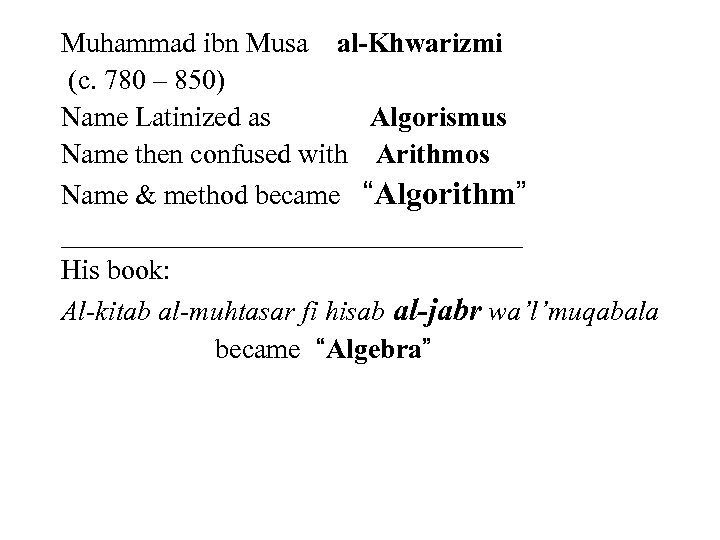Muhammad ibn Musa al-Khwarizmi (c. 780 – 850) Name Latinized as Algorismus Name then confused with Arithmos Name & method became “Algorithm” _________________ His book: Al-kitab al-muhtasar fi hisab al-jabr wa’l’muqabala became “Algebra”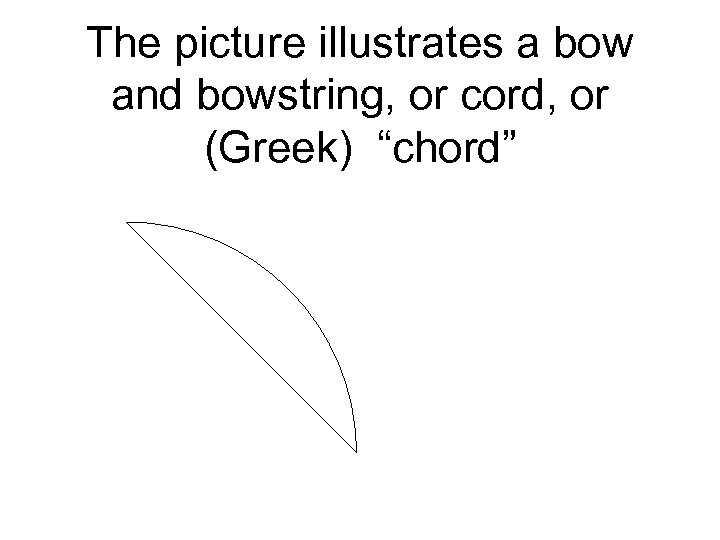The picture illustrates a bow and bowstring, or cord, or (Greek) “chord”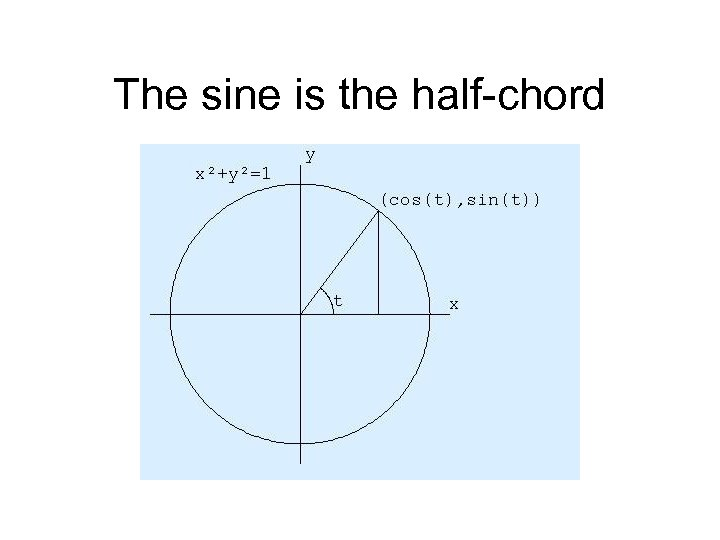The sine is the half-chord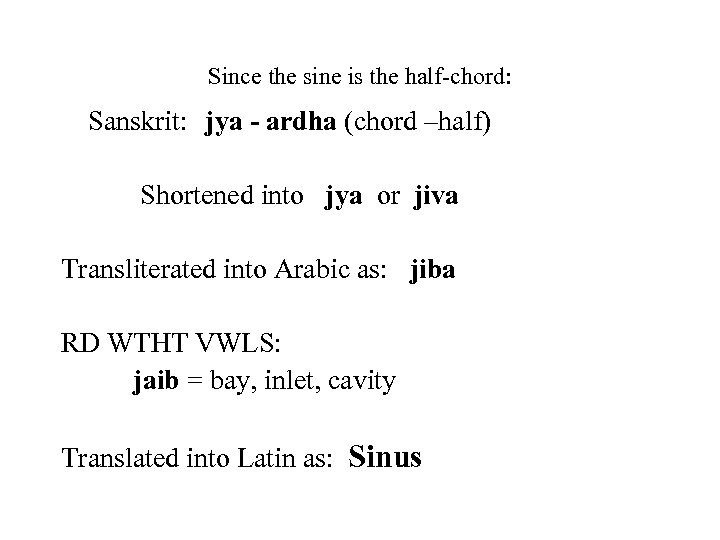Since the sine is the half-chord: Sanskrit: jya - ardha (chord –half) Shortened into jya or jiva Transliterated into Arabic as: jiba RD WTHT VWLS: jaib = bay, inlet, cavity Translated into Latin as: Sinus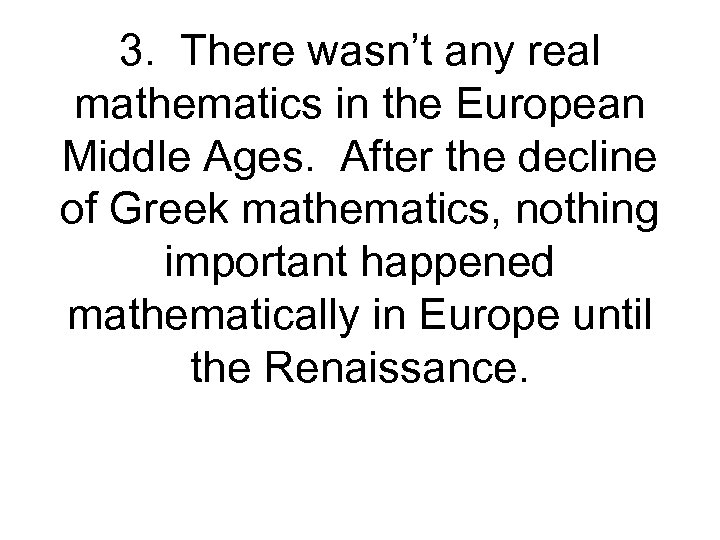3. There wasn’t any real mathematics in the European Middle Ages. After the decline of Greek mathematics, nothing important happened mathematically in Europe until the Renaissance.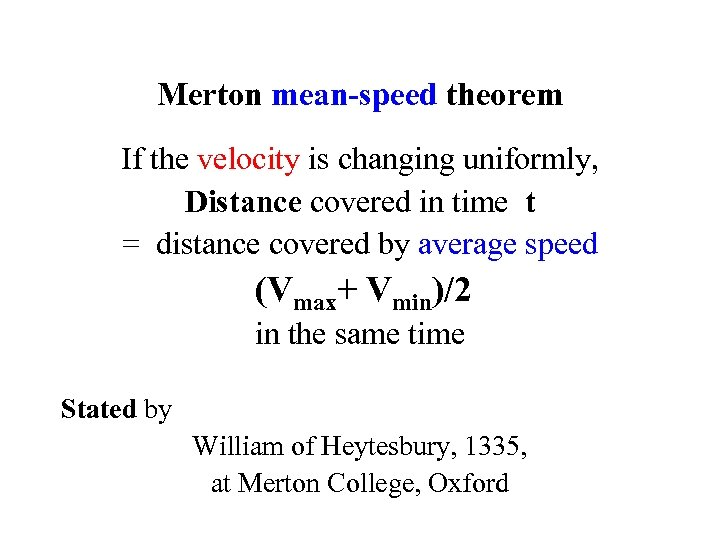Merton mean-speed theorem If the velocity is changing uniformly, Distance covered in time t = distance covered by average speed (Vmax+ Vmin)/2 in the same time Stated by William of Heytesbury, 1335, at Merton College, Oxford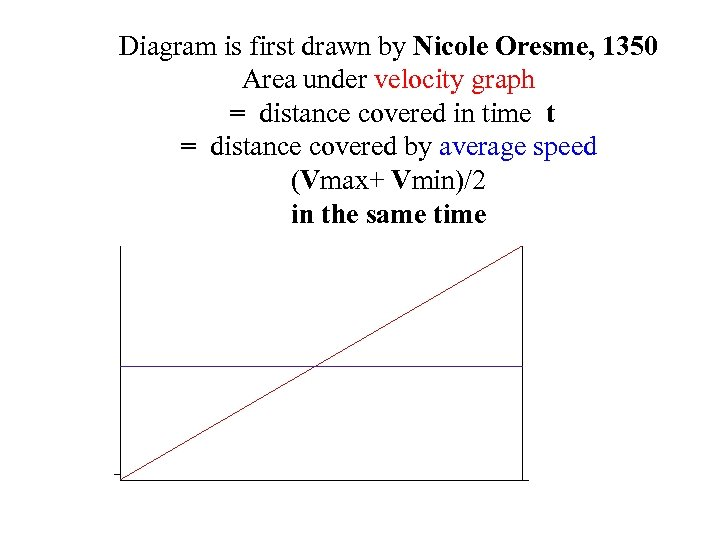Diagram is first drawn by Nicole Oresme, 1350 Area under velocity graph = distance covered in time t = distance covered by average speed (Vmax+ Vmin)/2 in the same time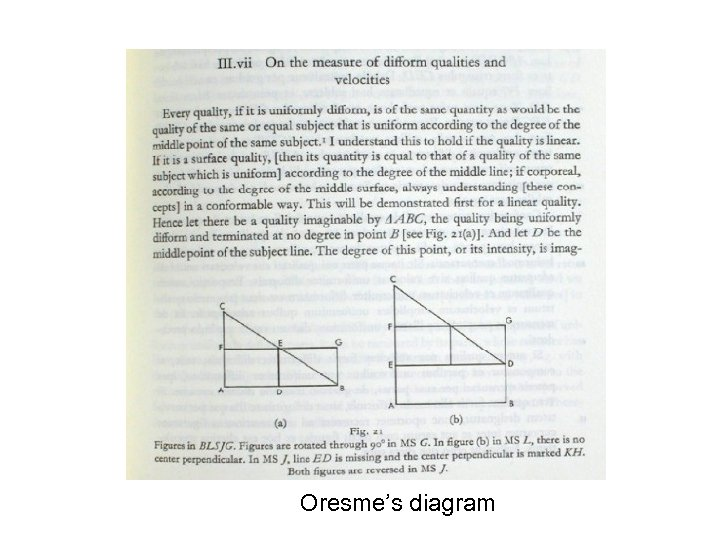Oresme’s diagram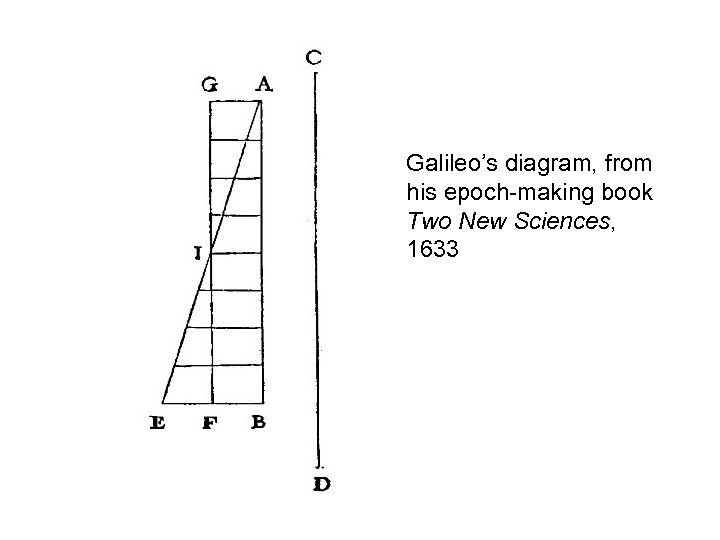Galileo’s diagram, from his epoch-making book Two New Sciences, 1633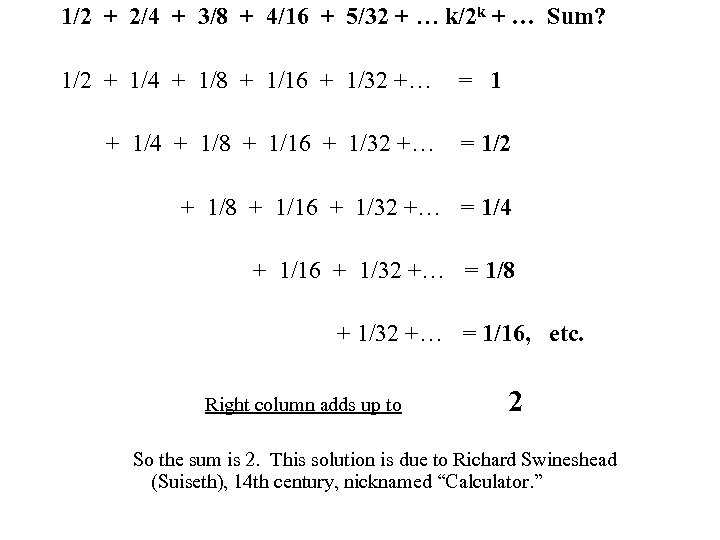1/2 + 2/4 + 3/8 + 4/16 + 5/32 + … k/2 k + … Sum? 1/2 + 1/4 + 1/8 + 1/16 + 1/32 +… = 1/2 + 1/8 + 1/16 + 1/32 +… = 1/4 + 1/16 + 1/32 +… = 1/8 + 1/32 +… = 1/16, etc. Right column adds up to 2 So the sum is 2. This solution is due to Richard Swineshead (Suiseth), 14 th century, nicknamed “Calculator. ”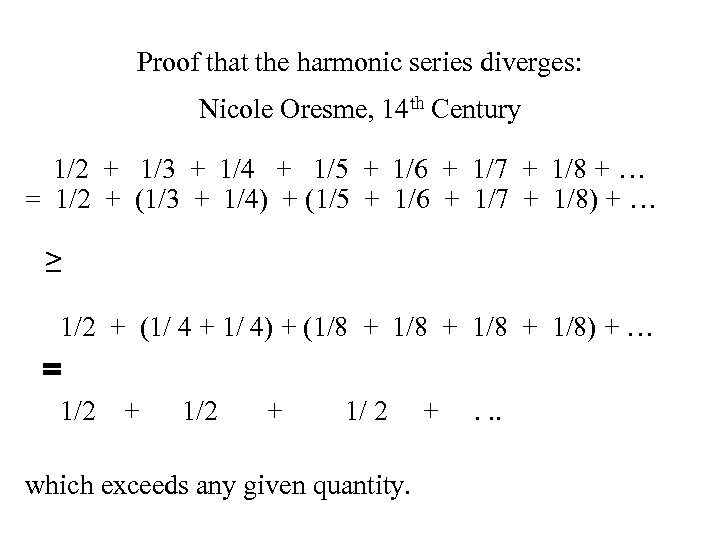Proof that the harmonic series diverges: Nicole Oresme, 14 th Century 1/2 + 1/3 + 1/4 + 1/5 + 1/6 + 1/7 + 1/8 + … = 1/2 + (1/3 + 1/4) + (1/5 + 1/6 + 1/7 + 1/8) + … ≥ 1/2 + (1/ 4 + 1/ 4) + (1/8 + 1/8) + … = 1/2 + 1/ 2 which exceeds any given quantity. + . . .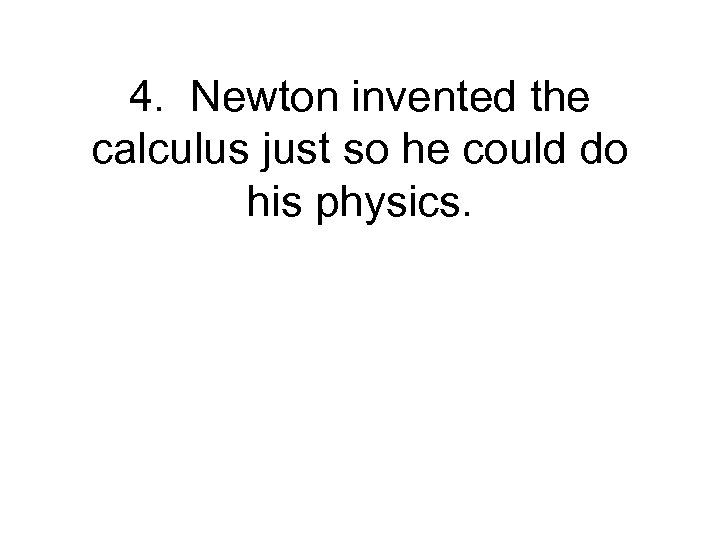4. Newton invented the calculus just so he could do his physics.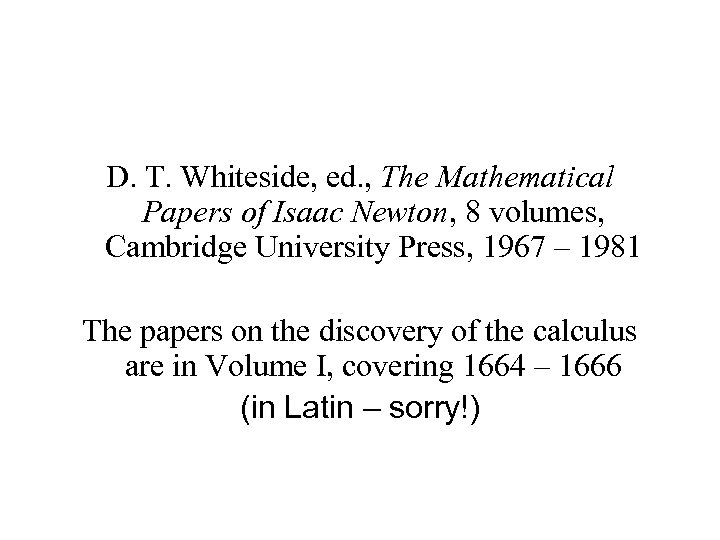D. T. Whiteside, ed. , The Mathematical Papers of Isaac Newton, 8 volumes, Cambridge University Press, 1967 – 1981 The papers on the discovery of the calculus are in Volume I, covering 1664 – 1666 (in Latin – sorry!)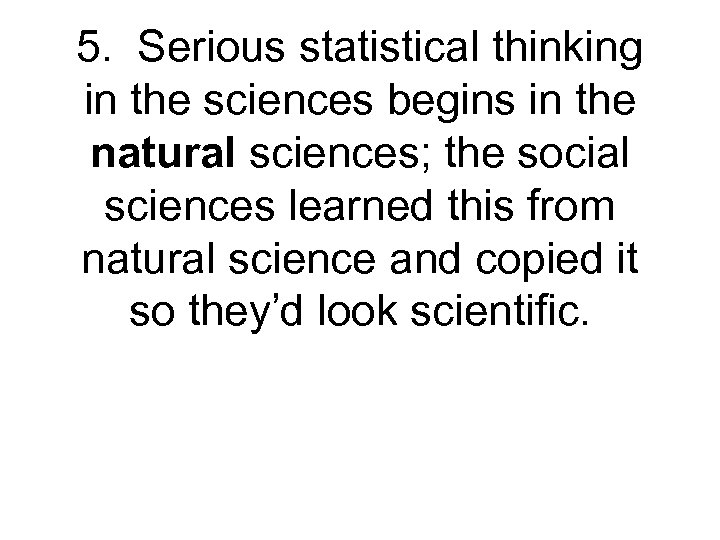5. Serious statistical thinking in the sciences begins in the natural sciences; the social sciences learned this from natural science and copied it so they’d look scientific.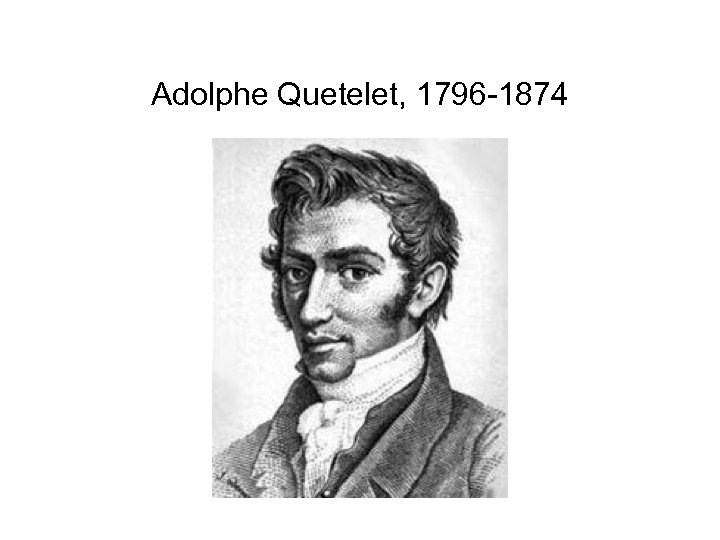Adolphe Quetelet, 1796 -1874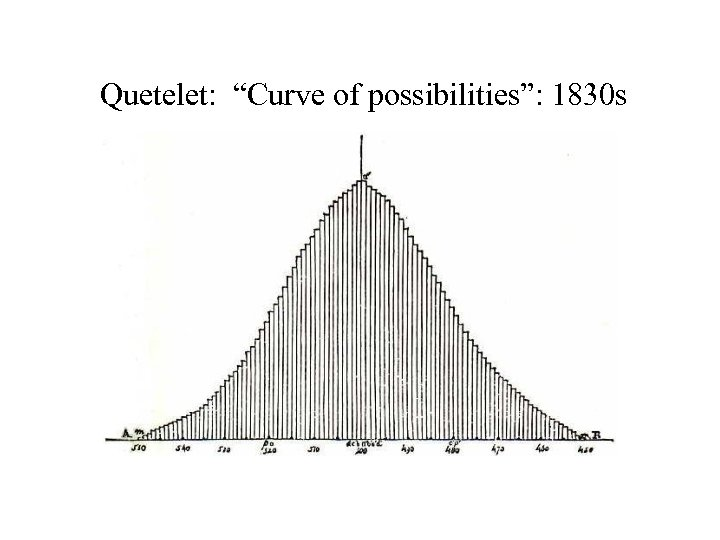Quetelet: “Curve of possibilities”: 1830 s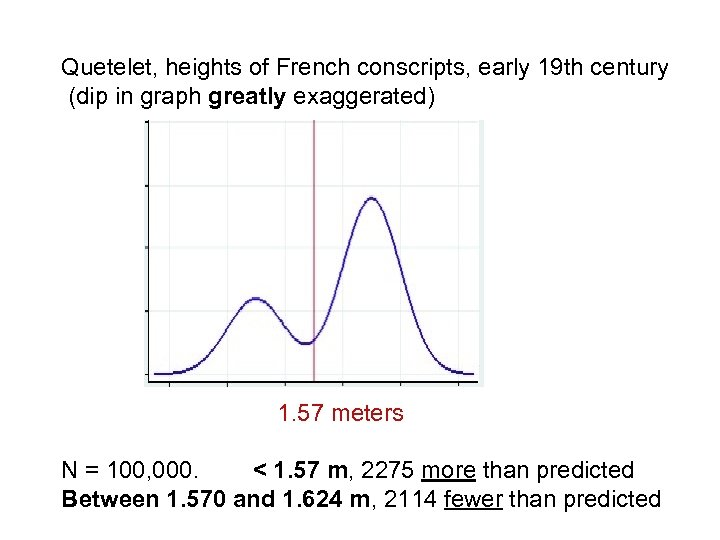Quetelet, heights of French conscripts, early 19 th century (dip in graph greatly exaggerated) 1. 57 meters N = 100, 000. < 1. 57 m, 2275 more than predicted Between 1. 570 and 1. 624 m, 2114 fewer than predicted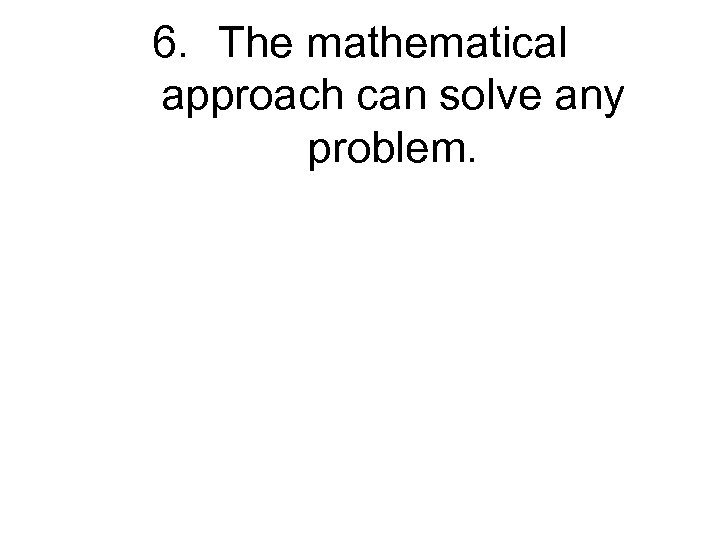6. The mathematical approach can solve any problem.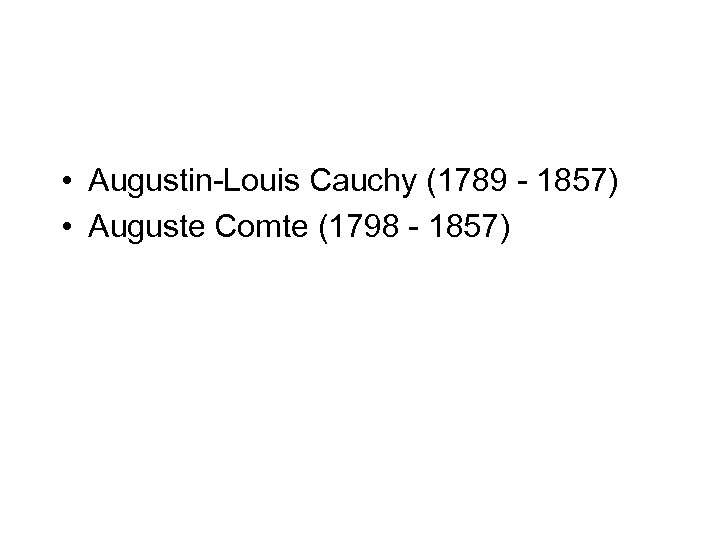• Augustin-Louis Cauchy (1789 - 1857) • Auguste Comte (1798 - 1857)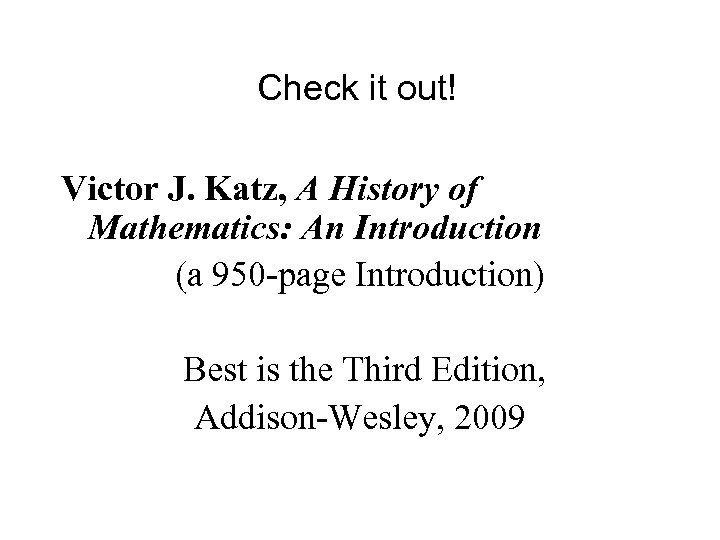Check it out! Victor J. Katz, A History of Mathematics: An Introduction (a 950 -page Introduction) Best is the Third Edition, Addison-Wesley, 2009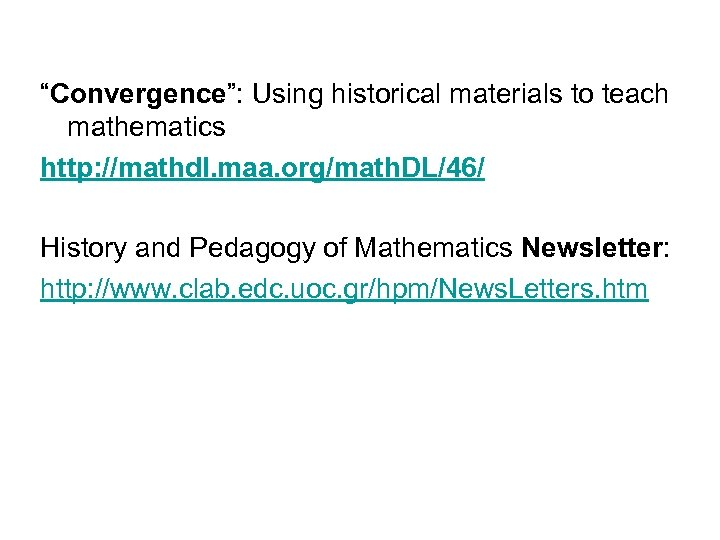“Convergence”: Using historical materials to teach mathematics http: //mathdl. maa. org/math. DL/46/ History and Pedagogy of Mathematics Newsletter: http: //www. clab. edc. uoc. gr/hpm/News. Letters. htm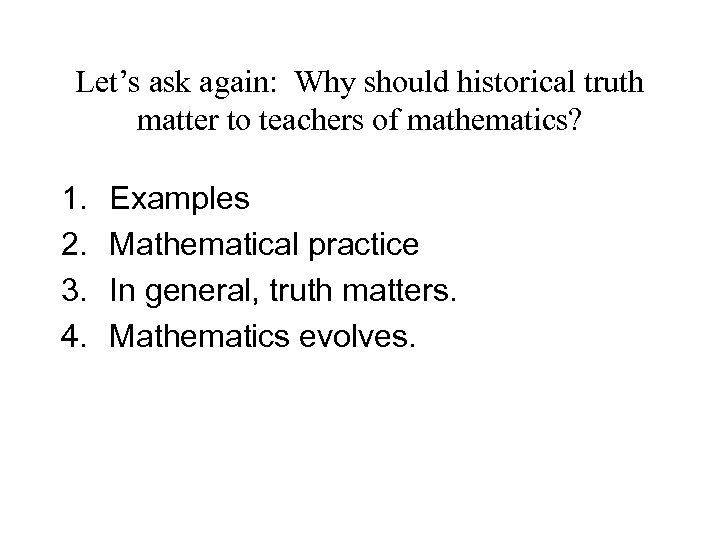Let’s ask again: Why should historical truth matter to teachers of mathematics? 1. 2. 3. 4. Examples Mathematical practice In general, truth matters. Mathematics evolves.